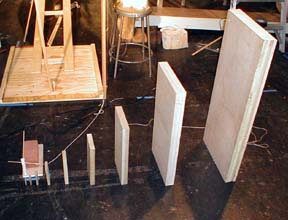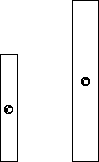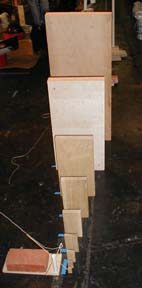Exponential Domino Fall

Exponential growth is amazing!Nine dominos each one 50% larger in all dimensions.

Introduction

A falling domino knocks over a larger domino, which in turn knocks over an even larger domino. This exponentially growing series can start with a normal domino and eight dominos later knock over a waist high domino with 10,000 times the mass and a million times the energy of the first one.Each domino is 1.5 times wider, higher, and thicker than its predecessor.

Material/Assembly

Each Domino should be 1.5 times higher, 1.5 times wider and 1.5 times thicker than the previous one.

I used a table saw to cut them out so that they had good flat bottoms.

The dominos that were made from sandwiches of wood were screwed together with wood screws. I used one wood screw at each corner placing two on one side of the boar and two on diagonal opposite corners on the other side.

#1 0.18 x 0.75" x 1.5" Use 3/16 inch thick stock.

#2 0.25" x 1.5" x 3" Use 1/4 inch plywood. (This is close to the size of a normal domino)

#3 0.375 x 2" x 4.5" Use 3/8 inch plywood

#4 0.5" x 3" x 6" Use 1/2 inch plywood

#5 0.75" x 4.5" x 9" Use 3/4" plywood or "1 by" pine shelving.

#6 1" x 6" x 12" Make a sandwich of 3/4 " plywood and 1/4" plywood

#7 1.5" x 9" x 18" Make a sandwich of 2 pieces of 3/4" plywood.

#8 2.25" x 14" x 28", Make a sandwich of 3 pieces of 3/4 inch plywood.

#9 3" x 21" x 42", Make a sandwich of 4 pieces of 3/4 inch plywood.

(My smallest 5 dominos were made on a milling machine from oak stock by Rienhardt.)

To Do and Notice

Place the largest domino first.

Place the next smallest domino so that the edge nearest the largest domino is one smaller domino width from the largest one.

Since the second largest domino is 14 inches wide, it is placed 14 inches from the largest domino.

Space each remaining domino a distance equal to its width from the next domino.

Knock over the smallest domino.

Notice how each domino knocks over its largest neighbor.

Notice also how each domino falls more slowly than it predecessor.

Listen to the sounds made by the falling dominos, they start with a gentle tic and end with a loud SLAM.

What's Going On?

One domino can knock over another simply by leaning against it.

However, one domino has some initial speed when it collides with its neighbor, this speeds up the reaction.

Math Root

Each Domino is 1.5 x 1.5 x 1.5 times the mass of the previous domino.

1.53 = 3.375

The next domino is 3.375 times heavier, so the third domino is 3.3752 = 11.4 times the mass of the first.

As an approximation every time you move two dominos the mass goes up by a factor of 10.

So the 9'th domino is 3.3758 = 16,800 times heavier than the first.

The energy of each domino when it falls is U = mgh where m is the mass, g is the acceleration of gravity, and h is the height the center of mass falls. Since the height of each domino is 1.5 times the previous one then the ninth domino falls with 16,800 x 1.58 = 2,200,000, more than two million times the energy of the first.

Go To Physics Root

The Growing Domino Fall was one link in a a Chain Reaction at the Exploratorium on Wednesday April 11, 2001.

The evening was the idea of Osher Fellow Arthur Ganson.

References

Scientific American, Amateur Scientist, Jearl Walker.

Falling Dominos